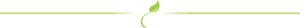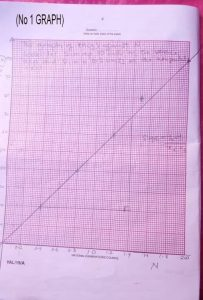HomeJambWaecNecoNabtebNews
Welccome To Examloaded.Com>>>>  Nigeria's No 1 Exam Expo Website, With 9 Years + of Experience Verified by Google #Na We Sure Pass!

HAVE YOU  PAID FOR YOUR WAEC NEXT SUBJECT   👇🏿👇🏿👇🏿

## WAEC DAILY RUNS PAYMENT WAEC RUNS - FINANCIAL ACCOUNTING   SMS/WHATSAPP: N1,000 MTN CARD Link/Password: N500 MTN CARD                                       Send the following through SMS: Exam name (eg. WAEC), Subject name, Card Pins And Your Phone number to 08162563540 ===============================Examloaded Answer Page: ExamAnswer.Net

This is Our Only Contact | Call | Text| WhatsApp## 08162563540Chat Admin After You Share To 20 People On Whatsapp to Redeem The Price

# 2019 Neco GCE Physics Practical | Expo Questions and Answers(1ai -1aiv)
S/N|M(g)|Real values of b(cm)|Converted values of b(cm)| Real Value of d(cm)| Converted values of d(cm)| N = b/d
1 |20 |5.30|26.50|12.9|64.5|0.41|
2 |40 |8.0 |40.0 |10 |50.0|0.80|
3 |60 |9.9 |49.5 |8.3|41.5|1.20|
4 |80 |11.0|55.0 |7.0|35.0|1.50|
5 |100|12.1|60.5 |6.0|30.0|2.0|

(1av)
{PLOT THE GRAPH}

(1avi)
Slope = Y2-Y1/X2-X1
=70-20/1.4-0.4
= 50/1
=50g

(1avii)
(i) I avoid error due to parallel when taking all my reading
(ii) I made sure that the meter rule be connect horizontally before taking my reading

(1bi)
(i) The total upward force must balance the downward.
(ii) The algebraic sum of all forces act on the body must be equal to zero.

(1bii)
The sum of clockwise moment = the sum of anti clockwise moment.
20 × (50-5) = 100 × (x-50)
20 × 45 = 100(x-50)
900 = 100x – 5000
900 + 5000 = 100x
5000/100 = 100x/100
x = 59cm

:. The distance from the balance point is 59-50 = 9cm.
:. 100g mass is 9cm from the balance point.

(3ai -3aiv)
S/N|R(ohms)|V(v)|I(A)|1/v(A/v)|R-¹(ohm-¹)|
1 | 1.0|1.21|1.80|1.49|1.00
2 | 2.0|1.50|1.50|1.00|0.50
3 | 3.0|1.64|1.36|0.83|0.33
4 | 4.0|1.72|1.29|0.75|0.25
5 | 5.0|1.76|1.23|0.70|0.20

(3av)
PLOT THE GRAPH

(3avi)
Slope = ΔA/V / ΔR-¹
= 1.28 – 0.80/ 0.80 – 0.30
= 0.48/0.50
= 0.96

Intercept (c) = 0.50

(3avii)
(i) I would ensure I made tight connection before taking my reading.
(ii) I would ensure I noted zero error on the voltmeter and Ammeter

(3bi)
Potential difference is the work done in moving a unit charge from a point in electric field to another. It has the unit of Volt.
V = work done/charge

(3bii)
r = 0.5ohms
I = 1.2A
R = ?
E = 3v
E = I(R+r)
E = IR + Ir
E – Ir = IR
R = E – Ir/I
= 3 – 1.2 × 0.5/1 .2
= 3 – 0.6/1.2
R = 2.4/1.2
R = 2 ohms